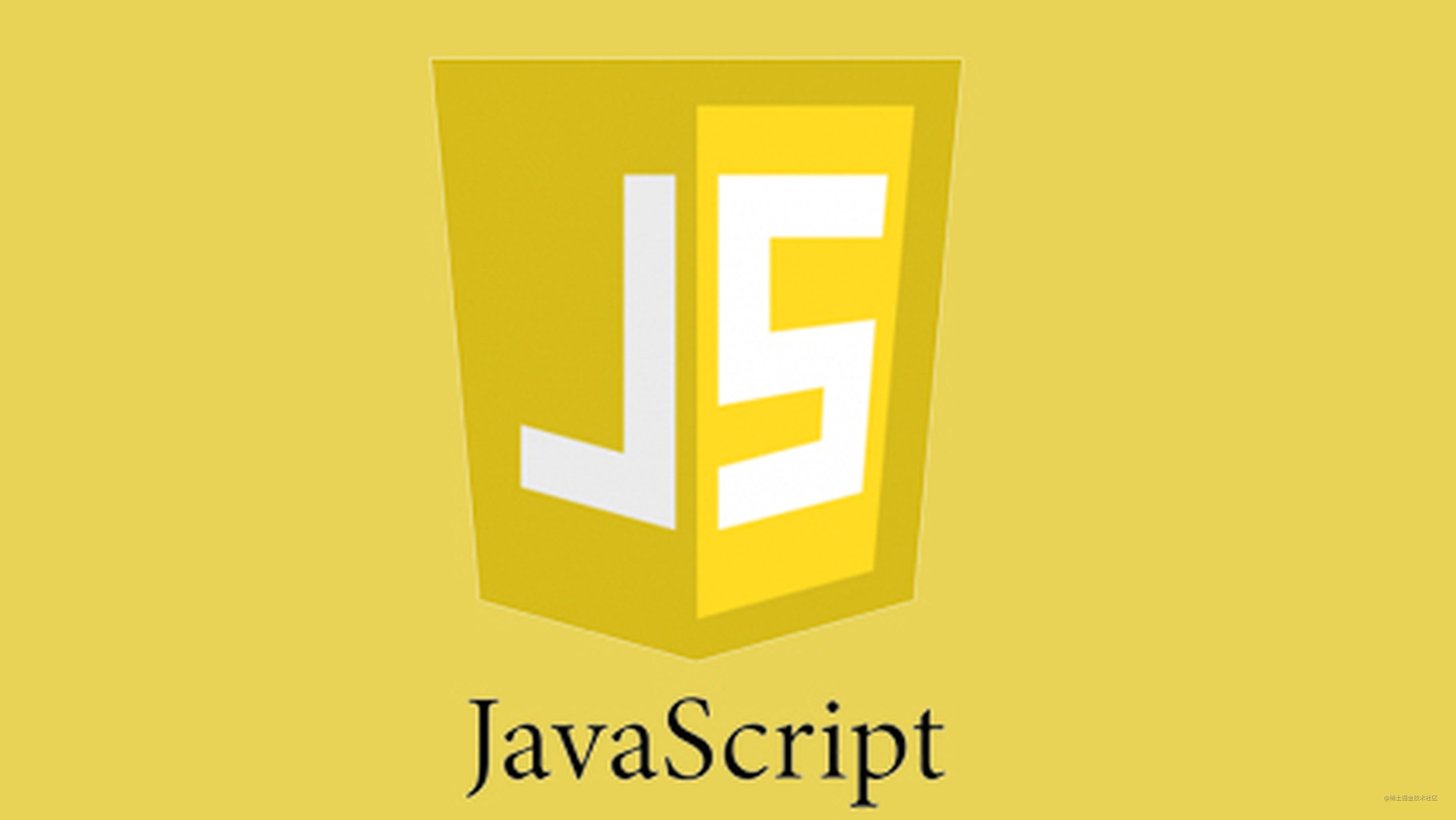# JavaScript 设计模式之观察者模式与发布订阅模式——维基百科

## 什么是观察者模式？

“老王，等你结婚了，记得叫我来喝喜酒！”

• 两者都是相互独立的对对象个体。
• 观察者在目标对象中订阅事件，目标广播发布事件。

• 老王就是模式中所谓的目标。
• 同学们在毕业前说的话就相当于在目标对象上订阅事件。
• 老王打电话通知朋友就是发布事件。
• 同学们各自作出了不同的行动回应。

1. 目标对象：Subject
2. 观察者对象：Observer

``````function Subject() {
this.observers = new ObserverList();
}
function ObserverList() {
this.observerList = [];
}
function Observer() {}

``````ObserverList.prototype.add = function(obj) {
return this.observerList.push(obj);
};

ObserverList.prototype.count = function() {
return this.observerList.length;
};

ObserverList.prototype.get = function(index) {
if (index > -1 && index < this.observerList.length) {
return this.observerList[index];
}
};

ObserverList.prototype.indexOf = function(obj, startIndex) {
var i = startIndex;

while (i < this.observerList.length) {
if (this.observerList[i] === obj) {
return i;
}
i++;
}
return -1;
};

ObserverList.prototype.removeAt = function(index) {
this.observerList.splice(index, 1);
};

};

Subject.prototype.removeObserver = function(observer) {
this.observers.removeAt(this.observers.indexOf(observer, 0));
};

``````Subject.prototype.notify = function(context) {
var observerCount = this.observers.count();
for (var i = 0; i < observerCount; i++) {
this.observers.get(i).update(context);
}
};

``````function Observer() {
this.update = function() {
// ...
};
}

``````function ObserverList() {
this.observerList = [];
}

return this.observerList.push(obj);
};

ObserverList.prototype.count = function() {
return this.observerList.length;
};

ObserverList.prototype.get = function(index) {
if (index > -1 && index < this.observerList.length) {
return this.observerList[index];
}
};

ObserverList.prototype.indexOf = function(obj, startIndex) {
var i = startIndex;

while (i < this.observerList.length) {
if (this.observerList[i] === obj) {
return i;
}
i++;
}
return -1;
};

ObserverList.prototype.removeAt = function(index) {
this.observerList.splice(index, 1);
};

function Subject() {
this.observers = new ObserverList();
}

};

Subject.prototype.removeObserver = function(observer) {
this.observers.removeAt(this.observers.indexOf(observer, 0));
};

Subject.prototype.notify = function(context) {
var observerCount = this.observers.count();
for (var i = 0; i < observerCount; i++) {
this.observers.get(i).update(context);
}
};

// The Observer
function Observer() {
this.update = function() {
// ...
};
}

## 什么是发布订阅模式？

``````var pubsub = {};
(function(myObject) {
var topics = {};
var subUid = -1;

myObject.publish = function() {};

myObject.subscribe = function() {};

myObject.unsubscribe = function() {};
})(pubsub);

``````topics = {
topicA: [
{
token: subuid,
function: func
}，
...
],
topicB: [
{
token: subuid,
function: func
}，
...
],
...
}

``````myObject.subscribe = function(topic, func) {
//如果不存在相应事件就创建一个
if (!topics[topic]) {
topics[topic] = [];
}
//将订阅对象信息记录下来
var token = (++subUid).toString();
topics[topic].push({
token: token,
func: func
});
//返回订阅者标识，方标在取消订阅的时候使用
};

``````myObject.unsubscribe = function(token) {
for (var m in topics) {
if (topics[m]) {
for (var i = 0, j = topics[m].length; i < j; i++) {
if (topics[m][i].token === token) {
topics[m].splice(i, 1);
}
}
}
}
return this;
};

``````myObject.publish = function(topic, args) {
if (!topics[topic]) {
return false;
}
var subscribers = topics[topic],
len = subscribers ? subscribers.length : 0;
while (len--) {
subscribers[len].func(args);
}
return this;
};

``````var pubsub = {};

(function(myObject) {
var topics = {};
var subUid = -1;

myObject.publish = function(topic, args) {
if (!topics[topic]) {
return false;
}
var subscribers = topics[topic],
len = subscribers ? subscribers.length : 0;
while (len--) {
subscribers[len].func(args);
}
return this;
};

myObject.subscribe = function(topic, func) {
if (!topics[topic]) {
topics[topic] = [];
}
var token = (++subUid).toString();
topics[topic].push({
token: token,
func: func
});
};

myObject.unsubscribe = function(token) {
for (var m in topics) {
if (topics[m]) {
for (var i = 0, j = topics[m].length; i < j; i++) {
if (topics[m][i].token === token) {
topics[m].splice(i, 1);
}
}
}
}
return this;
};
})(pubsub);

## 二者的区别和联系

### 区别：

1. 观察者模式中需要观察者对象自己定义事件发生时的相应方法。
2. 发布订阅模式者在发布对象和订阅对象之中加了一个中介对象。我们不需要在乎发布者对象和订阅者对象的内部是什么，具体响应时间细节全部由中介对象实现。

### 联系：

1. 二者都降低了代码的耦合性。
2. 都具有消息传递的机制，以数据为中心的设计思想。

## 实战

``````var template = `<span><% this.value %></span>`;

``````var data = {
value: 0
};

``````setInterval(function() {
data.value++;
}, 1000);

``````setInterval(function() {
data.value++;
document.body.innerHTML = TemplateEngine(template, data);
}, 1000);

``````var template = `<span><% this.value %></span>`;
var data = {
value: 0
};
function render() {
document.body.innerHTML = TemplateEngine(template, data);
}
render();
pubsub.subscribe("change", render);
setInterval(function() {
data.value++;
pubsub.publish("change");
}, 1000);
};

1. 不同功能紧密耦合，如果以后要修改该功能，很可能牵一发而动全身。
2. 往往实际开发中我们的订阅者不止一个，发布者的消息也不止一个，远远比这个例子的逻辑复杂的多。剪不断，理还乱。

## 值得一提：事件监听的实现

``````subject.addEventListener("click", () => {
//...
});

-EFO-

2019/04/28

AJie